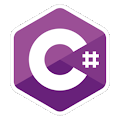Programming Course in C# ¡Free!

# Product

Proposed exercise

Create a program which asks the user for two integer numbers and displays their multiplication, but not using "*". You must use consecutive sums. (Hint: remember that 3*5 = 3+3+3+3+3 = 15)

Output

Solution

```using System;
public class Product
{
public static void Main()
{
Console.Write("Enter the first number: ");

Console.Write("Enter the second number: ");

int result=0;
int i=0;

while( i < n2 )
{
result = result+n1;
i++;
}

Console.WriteLine("{0} X {1} = {2}", n1, n2, result);
}
}
```